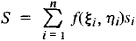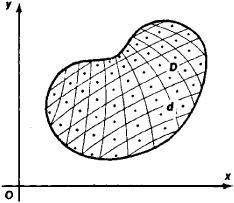# Multiple Integral

Also found in: Wikipedia.

## multiple integral

[′məl·tə·pəl ′int·ə·grəl]
(mathematics)
An integral over a subset of n-dimensional space.
McGraw-Hill Dictionary of Scientific & Technical Terms, 6E, Copyright © 2003 by The McGraw-Hill Companies, Inc.
The following article is from The Great Soviet Encyclopedia (1979). It might be outdated or ideologically biased.

## Multiple Integral

an integral of a function defined on some region in a plane and in three-dimensional or n -dimensional space. The corresponding multiple integrals are referred to as double integrals, triple integrals, and n-tuple integrals, respectively.

Let the function f(x, y ) be defined on some region D of the plane xOy. Let us divide D into n subregions di whose areas are equal to si, choose a point (ξi, ηi) in each subregion di (see Figure 1), and form the integral sumIf as the maximal diameter of the subregions d, decreases without bound the sums S have a limit independent of the choice of the points (ξi, ηi), then this limit is called the double integral of the function f(x, y) over the region D and is denoted by

∫ ∫Df (x,y) ds

A triple integral and, in general, an n -tuple integral are defined analogously.Figure 1

In order for the double integral to exist, it is sufficient that, for example, the region D be a closed (Jordan) measurable region and that the function f(x, y) be continuous throughout D. Multiple integrals possess a number of properties similar to those of ordinary integrals. In order to calculate a multiple integral we reduce it to an iterated integral. Green’s formulas and the Green-Ostrogradskii theorem can be used in special cases to reduce multiple integrals to integrals of lower dimension. Multiple integrals find wide application. Volumes of bodies, as well as masses, static moments, and moments of inertia ( of bodies, for example) are expressed using multiple integrals.

References in periodicals archive ?
Since the integral is only conditionally convergent, we have to pay attention to the order in which we calculate the multiple integral. In this case, our goal is to integrate sequentially the shells around the test mass, starting from r = 0 and extending to r = to, thus we have to integrate first over the variables 9 and 0 and only then over r.
Kiss, "Stability analysis of linear systems with interval time-varying delays utilizing multiple integral inequalities," Applied Mathematics and Computation, vol.
The third method was direct integration , which has encountered mathematical difficulties in the multiple integral and quantitative description of computational accuracy.
When doing change of variables in a multiple integral we need to know the Jacobian.
Schlafli, On the multiple integral [[integral].sup.n] dxdy *** dz whose limits are [p.sub.1] = [[alpha].sub.1] x + [b.sub.1] y + *** + [h.sub.1] z > 0, [p.sub.2] > 0, ..., [p.sub.n] > 0 and [x.sup.2] + [y.sup.2] + *** + [z.sup.2] < 1, in Gessammelte Mathematischen Abhandlungen, Band II, Birkhaauser, Basel, 1953, pp.
As part of the 2012 startup project the turbo blower control system required upgrading, and Cordell provided the project management, design, manufacture testing and, installation & commissioning resources on multiple integral elements.
Typically, the measure of the average impact of these variables could be reduced to a multiple integral of a relatively complex integrand function after a lengthy derivation .
Tseng, On certain multiple integral inequalities related to Hermite-Hadamard inequalities, Utilitas Math.
The multi-time cost functional can be introduced either using a path independent curvilinear integral, or using a multiple integral. Using a similar argument as in , page 144, variational problems with multiple integrals can be converted to problems with curvilinear integrals and conversely.
Recently, a new multiple integral inequality was introduced following a similar line as in proof of the Jensen inequality in , and a novel delay-dependent stability criterion was established, which has unfortunately observed that the computational burden is slightly heavy.
Tseng, On certain multiple integral inequalities related to Hermite-Hadamard inequality, Utilitas Mathematica 62(2002), 131-142.
The multiple integral I([t.sub.0]) suggests to introduce a new coordinate

Site: Follow: Share:
Open / Close